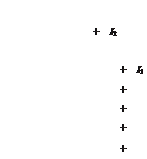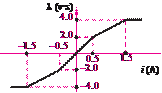### Create an Account

Home / Questions / A single loop of wire carrying current I2 is placed near the end of a solenoid having N tu...

# A single loop of wire carrying current I2 is placed near the end of a solenoid having N turns and carrying current I1 as shown in Figure P132 The solenoid is fastened to a horizontal surface but

A single loop of wire carrying current I2 is placed near the end of a solenoid having turns and carrying current I1, as shown in Figure P13.2. The solenoid is fastened to a horizontal surface, but the single coil is free to move. With the currents directed as shown, is there a resultant force on the single coil? If so, in what direction? Why?

inductor.

13.6      Repeat Problem 13.5 if

λ2

 +

= 0.5     λ2

13.7      An iron-core inductor has the characteristic shown in Figure P13.7:

a.    Determine the energy and the incremental inductance for = 1.0 A.

b.    Given that the coil resistance is 2 and that

i(t) = 0.5 sin 2πt, determine the voltage across the terminals of the inductor.Figure P13.2

Jun 18 2020 View more View LessSubscribe To Get Solution